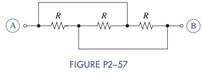Create an Account

Home / Questions / Find the equivalent resistance between terminals A and B in Figure P2−57.

Find the equivalent resistance between terminals A and B in Figure P2−57.

Find the equivalent resistance between terminals A and B in Figure P2−57.Jul 28 2020 View more View LessSubscribe To Get Solution